# Stabilized Nodal Strain Tetrahedron for Almost Incompressible Materials

André Hemmler did his honours project at the Department of Mechanics & High Performance Computing (TUM) and
was supervised by Prof. Dr.-Ing. Michael W. Gee.

### Introduction

Descretizing complex three-dimensional structures, tetrahedral elements have a decisive advantage compared to hexahedral elements. However, classical linear tetrahedral elements have numerous other weaknesses. For incompressible or nearly incompressible materials, the most severe shortcoming is volumetric locking, i.e. tetrahedral elements behave much too stiff. Unacceptably fine discretization may be necessary to obtain adequate results. In contrast to shear locking or membrane locking which are geometrical locking effects, volumetric locking is a material locking effect which gets significant for Poisson ratios ν → 0.5, i.e. for almost incompressible materials. Volumetric locking is caused by the fact that finite elements (such as linear tetrahedral elements) are not able to meet the kinematic incompressibility condition: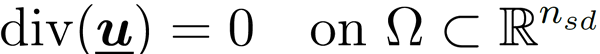Too many deformation constraints are imposed on the solution and the discretized structure behaves too stiff. Special techniques have been developed to avoid volumetric locking. The best known ones are:

• higher order interpolation functions
• reduced or selective reduced integration
• mixed element formulations with additional pressure-unknowns

In this honours project a new tetrahedral element formulation is introduced which is based on a nodal strain formulation in conjunction with a classical element formulation. The numerical stability of the proposed formulation is investigated and its limits are presented.

### Stabilized nodal strain formulation of tetrahedral elements

The nodal strain formulation is based on a nodally averaged strain and stress tensor according to . The volume based averaging procedure of the nodal values is performed over the patch SelI of elements adjacent to node I (see figure 1). This formulation leads to a relaxation of the incompressibility constraints and hence is free of the volumetric locking phenomenon. However, as this nodal strain technique leads to an instable formulation, a stabilization scheme depending on a classical displacement-based element formulation is used. For the stabilization term, a center node for each tetrahedron is introduced and the element is divided into four subelements. On this finer mesh, element based integration is performed. This stabilization is restricted to the deviatoric component to avoid the reintroduction of volumetric locking by the elementwise integration of the linear tetrahedral elements. Consequently, the discretized weak form of the proposed formulation is given by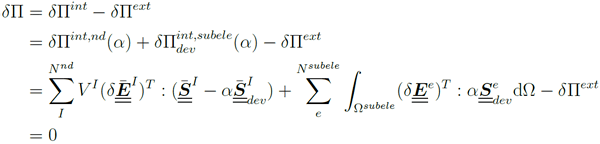where α with 0 < α ≤ 1 is the stabilization parameter, δE is the virtual Green-Lagrange strain tensor, S is the second Piola-Kirchhoff stress tensor and V is the averaging volume of the nodal strain formulation. The superscripts (.)int and (.)ext describe the internal and the external component of the virtual work and the superscripts (.)nd and (.)subele denote the parts evaluated by the nodal strain formulation and the parts evaluated by the classical element based formulation on the subelements, respectively.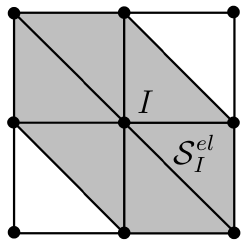Fig. 1: Two-dimensional visualization of the element patch SelI of the nodal strain formulation.

### Numerical example – Cook’s membrane

Cook’s membrane (see figure 2) is a popular benchmark problem for volumetric locking of nearly incompressible materials.

#### Convergence behaviour

In figure 2 the convergence behaviour of the maximum tip displacement for different elements under mesh refinement is considered. The locking-free 8-noded trilinear hexahedral element (hex8) with 24 internal strain degrees of freedom (enhanced assumed strain method) can be considered as reference. The 4-noded displacement-based terahedron (tet4) obviously suffers under volumetric locking. The elements behave much too stiff for coarse discretizations. The proposed stabilized nodal strain formulation (NStet5(α)) shows a much better convergence behaviour close to the one of the hex8-element. However, reducing the deviatoric stabilization (α → 0) the instable characteristic of the formulation reflects in an asymptotic deviation to the reference solution of the hex8-element.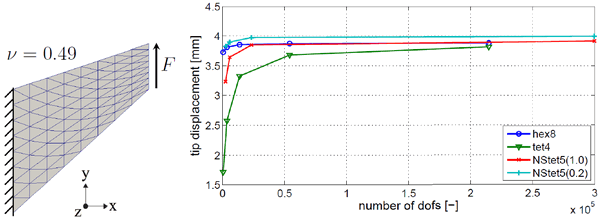Fig. 2: Geometry of Cook’s membrane problem (left) and convergence plot (right) of the tip displacement max(│u│) under mesh refinement for the 8-noded trilinear hexahedral element (hex8) with an enhanced assumed strain method, the standard 4-noded terahedron (tet4), the stabilized nodal strain formulation with α=1.0 (NStet5(1.0)) and the stabilized nodal strain formulation with α=0.2 (NStet5(0.2)).

#### Eigenvalue analysis

The eigenvalue analysis of Cook’s membrane problem without supports gives a better insight to the instable behaviour of the nodal strain elements. For α → 0 (see figure 3) the eigenvalues (nλ > 6) tend towards zero, i.e. the stiffness matrix is almost singular. This observation is illustrated in the plots of the eigenmodes of the discretized structure (see figure 4). Reducing the stabilization (α → 0), non-physical checkerboard modes get dominant and surpass the physical eigenmodes.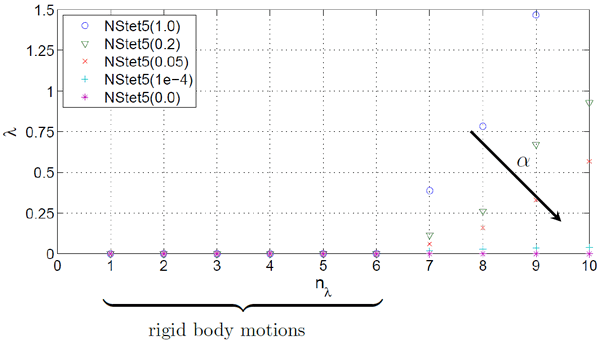Fig. 3: Eigenvalues of global stiffness matrix for NStet5(α)-elements with different stabilization factors α for Cook’s membrane problem (without supports).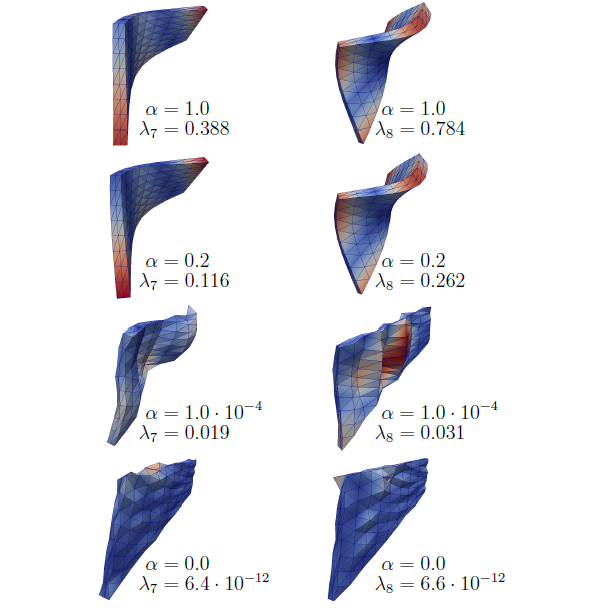Fig. 4: Eigenmode 7 (left) and eigenmode 8 (right) for α=1.0, α=0.2, α=1.0 · 10-4 and α=0.0 (from top to bottom) for Cook’s membrane problem (without supports).

### Conclusions

The instable behaviour of the nodal strain formulation can only be overcome by a fully deviatoric stabilization (α=1.0), i.e. the total deviatoric part has to be expressed in the classical displacement-based formulation on the subelements. This results in a formulation that is closely related to the F-bar-based element formulation of Souza Neto et al. . Alternatively, higher order elements could be used in combination with the nodal strain formulation. The so called MINI-element  is the simplest upgrading of the linear tet4-element and is frequently used in mixed finite element methods for incompressible materials. It uses an additional bubble node in the centroid of the tetrahedron.

### References

  M. W. Gee, C. R. Dohrmann, S. W. Key, and W. A. Wall. A uniform nodal strain tetrahedron with isochoric stabilization. International journal for numerical methods in engineering., 78, 2009.  C. R. Dohrmann, M. W. Heinstein, J. Jung, S. W. Key, and W. R. Witkowski. Node-based uniform strain elements for three-node triangular and four-node tetrahedral meshes. International Journal for Numerical Methods in Engineering, 47, 2000.  E. A. de Souza Neto, F. M. Pires, and D. R. J. Owen. F-bar-based linear triangles and tetrahedra for finite strain analysis of nearly incompressible solids. part I: formulation and benchmarking. International Journal for Numerical Methods in Engineering, 62, 2005.  D. N. Arnold, F. Brezzi, and M. Fortin. A stable finite element for the stokes equations. Calcolo, 21, 1984.